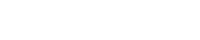As student pilots, we all remember learning how to use a chart to determine the crosswind component of the wind, based on the wind velocity and the angle between the wind direction and the runway. That's great for a table-top exercise, but can be a little cumbersome when actually flying the airplane. (See Figure 1) Here's a quick and easy way to do it in your head, without the need to bring out your crosswind component chart. (Warning: there's a little bit of mental math involved, but nothing severe.)

As you approach the runway, any wind can be thought of as having two components: headwind and crosswind. (See Figure 2) Crosswind is the component that pushes us to one side or the other, and is what we have to compensate for. The angle between the actual wind direction and the runway determines the size/strength of the crosswind component. This is expressed as a fraction between 0 and 1. Just multiply that fraction times the wind and you have your crosswind component. Examples and a table of fractions are below.

For those of you who are more mathematically inclined, here's the formula:

Let's call the angle of the wind to the runway Theta (Θ), the wind speed V and the crosswind component CW. (See Figure 3) CW = V * SIN (Θ)

You can easily determine the trigonometric Sine of an angle using your Windows or phone calculator – just go to the Scientific View. But that's the hard way.

In Figure 4 , we've done the trig for you. There is a table of angles and multipliers that you can print and put on your kneeboard or partially memorize. Just remember that a 10-degree angle results in a 1/6 multiplier, a 20-degree angle a 1/3 multiplier, and so forth. For Example, a 15-knot wind that is 20 degrees off the runway heading gives you a crosswind component of 5 knots. (15 x 1/3 = 5) (Realistically, any wind beyond 60 degrees off the runway heading should be considered as all crosswind.)

Still nervous about crosswind landings? Then wait for the right conditions, get with your favorite CFI, and go out and do some!

You might be interested in: